# Simplified Independent Complex Samples T-Test - Comparing Two Proportions

Where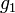$g_1$ and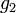$g_2$ are the two proportions,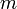$m$ and$n$ are their respective sample sizes and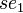$se_1$ and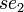$se_2$ are their respective Standard Errors: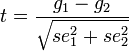$t=\frac{g_1-g_2}{\sqrt{se^2_1 + se^2_2}}$,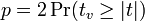$p = 2\Pr(t_v \ge |t|)$, and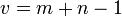$v = m + n - 1$.